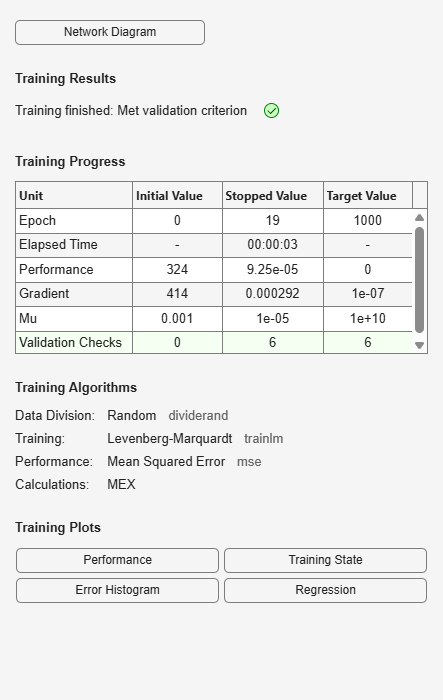# plotregression

Plot linear regression

## Syntax

``plotregression(targets,outputs)``
``plotregression(targs1,outs1,'name1',targs2,outs2,'name2',...)``

## Description

example

````plotregression(targets,outputs)` plots the linear regression of `targets` relative to `outputs`. ```
````plotregression(targs1,outs1,'name1',targs2,outs2,'name2',...)`generates multiple plots.```

## Examples

collapse all

This example shows how to plot the linear regression of a feedforward net.

```[x,t] = simplefit_dataset; net = feedforwardnet(10); net = train(net,x,t); y = net(x); plotregression(t,y,'Regression')```## Input Arguments

collapse all

Network targets, specified as a matrix or cell array.

Network outputs, specified as a matrix or cell array.